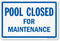This guide covers how you should break Chlorine Lock. Chlorine lock isn't the end of the world but it is a common problem for swimming pool owners. Chlorine lock means that the chlorine in the pool is useless which means the water isn't being sanitized. It can also indicate the presence of chloramines which give off a chlorine smell. Below are a few ways to deal with this issue.

1. How does chlorine lock occur? Chlorine lock can occur when there is too much cyanuric acid (also referred to as conditioner or stabilizer) in the water. This occurs when too much stabilizer is added to the water or when the swimming pool isn't being partially drained and refilled periodically. Chlorine lock can also occur if the pH is unbalanced. The quickest way to determine if a chlorine lock is present is to perform a test for total chlorine and free chlorine. Total chlorine is a measure of all the chlorine in the water and free chlorine is the chlorine that actually sanitizes the water. The two results should equal each other under normal conditions. if they don't you likely have chlorine lock. For example a free chlorine reading of 3 and a total chlorine reading of 3 is fine. If you have a free chlorine reading of 3 and a total chlorine reading of 7, there is a problem.

2. There have been many discussions questioning the existence of chlorine lock and how to resolve it. While there are many ways to resolve a chlorine lock, I'll only be covering a few. The first method is to partially drain and refill the pool. Using this method will take some time depending on how badly the chlorine is locked. There is no math equation for this method. You simply need to partially drain, refill, retest and repeat (if necessary).

3. Another way to break chlorine lock is to shock the pool with a non-chlorine shock. Non-chlorine shock will oxidize the chlorine in the swimming pool. To determine the amount of non-chlorine shock you will need, you will need to do some math. You will need to subtract the free chlorine from the total chlorine and multiply that by the number of gallons in the pool divided by 10,000 then multiply by 2. To make it easy it looks like this: (TC-FC) x (#of gallons/10,000) x 2 = amount of non-chlorine shock. If we use a total chlorine reading of 5 ppm and a free chlorine of 3 ppm on a 15,000 gallon pool, the equation would look like this: (5-3) x (15,000/10,000) x 2 = x. Therefore: 2 x 1.5 x 2 = 6. I would need to add 6 lbs. of a non-chlorine shock to the pool.

4. Yet another way to resolve a chlorine lock is to reach what is called breakpoint chlorination. Breakpoint chlorination is the point where the chemical bond that ties nitrogen, chlorine and ammonia together by using large amounts of chlorine. This will cause the chlorine residual to drop rapidly. Reaching a true chlorine breakpoint is vital to breaking a chlorine lock. If breakpoint is not reached using this method, the problem will only get worse. To accomplish a breakpoint, 7.6 free chlorine molecules are used to break apart an individual combined chlorine molecule. The amount of product you will need will vary depending on the type of shock you are using. Aside from knowing your total chlorine, free chlorine, number of gallons in the pool, and the weight of a gallon of water (8.34 lbs.), you will also need to know the type of pool shock you are using. Before attempting this method, the pH needs to be between 7.2-7.4. This equation will be a two part process. The first step will be determining the ppm of chlorine needed to reach breakpoint: (TC-FC) x 10. Using the same amount above, the equation would look like this: (5-3) x 10 = x ppm or 2 x 10 = 20 ppm. I will need 20 ppm of chlorine to reach breakpoint in this 15,000 gallon pool. The next step is to determine how much product I will need. I'm using calcium hypochlorite in this example and need 2 oz. per 10,000 for a 1 ppm increase. I'll need to multiply 2 oz. x (gallons in pool/10,000) x (ppm needed/ppm dosage) which simply put is: 2 oz. x (15,000/10,000) x (20 ppm/1 ppm) or 2 oz x 1.5 x 20= 60 oz. To convert ounces to pounds, I divide by 16: 60 oz./16= 3.75 lbs. So to reach breakpoint in my 15,000 gallon pool I will need 3.75 lbs. of calcium hypochlorite.

If you have any other questions about pool and spa products please do let us know - we are here to help!